Programs run sequentially from top to bottom, left to right. If you run the code along with a pencil, it will look like a thread. So the program execution flow is called a thread. So far, we have seen only single-threaded programs. In this article, I'll show you how to make a program that has more than two threads. Such a program is called a multi-thread program.

You can create a multi-threaded program by extending the Thread class or implementing the Runnable interface.

```package net.java_school.thread.test;

public void doA() {
char[] alphabet = {'A','B','C','D','E','F','G','H','I','J'};
int length = alphabet.length;
for ( int i=0; i < length; i++ ) {
System.out.print(alphabet[i]);
}
}

public void doB() {
for (int i = 0; i < 10; i++) {
System.out.print(i);
}
}

public static void main(String[] args) {
st.doA();
st.doB();
}
}
```

DoA() and doB() are executed on the same thread. Therefore doB() is executed after doA() ends.

Let's turn our example into a multi-threaded program.

#### How to create a multi-threaded program by extending the Thread class

Create a class that extends the Thread class.
Override the run() method of the Thread class and make doA() and doB() methods in the class's body like below.

```package net.java_school.thread.test;

public void doA() {
char[] alphabet = {'A','B','C','D','E','F','G','H','I','J'};
int length = alphabet.length;
for ( int i = 0; i < length; i++ ) {
System.out.print(alphabet[i]);
try {
//Take 0.01 seconds to allow another thread to take control.
} catch (InterruptedException e) {
e.printStackTrace();
}
}
}

public void doB() {
for (int i = 0; i < 10; i++) {
System.out.print(i);
try {
//Take 0.01 seconds to allow another thread to take control.
} catch (InterruptedException e) {
e.printStackTrace();
}
}
}

@Override
public void run() {
doA();
}

public static void main(String[] args) {
mt1.start();
mt1.doB();
}
}
```

If you call the start() method after creating an object from this class, the JVM makes another thread and executes doA() called by the run() method on the thread. This thread is not where the method main is performed.

#### How to create a multi-threaded program by implementing the Runnable interface

Create a class that implements the Runnable interface.
Implement the run() method of the Runnable interface and make doA() and doB() methods in the interface's body like below.

```package net.java_school.thread.test;

public class MultiThread2 implements Runnable {

public void doA() {
char[] alphabet = {'A','B','C','D','E','F','G','H','I','J'};
int length = alphabet.length;
for ( int i = 0; i < length; i++ ) {
System.out.print(alphabet[i]);
try {
//Take 0.01 seconds to allow another thread to take control.
} catch (InterruptedException e) {
e.printStackTrace();
}
}
}

public void doB() {
for (int i = 0; i < 10; i++) {
System.out.print(i);
try {
//Take 0.01 seconds to allow another thread to take control.
} catch (InterruptedException e) {
e.printStackTrace();
}
}
}

@Override
public void run() {
doA();
}

public static void main(String[] args) {
t.start();
mt2.doB();
}
}
```

The highlighted part of the method main does the followings:

1. Create an instance of the Runnable interface type
2. Pass the runnable interface type instance as an argument to the constructor when creating a thread instance
3. Call the Thread's start() method

### Critical Section

A critical section is a piece of code that accesses a shared resource.
Let's look at an example of the problems if a programmer doesn't handle the critical section properly. In the bank example, change the withdraw() method of NormalAccount as shown below. The change increases the probability that the account's balance becomes negative.

```@Override
public void withdraw(double amount)  {
try {
} catch (InterruptedException e) {
e.printStackTrace();
}

if (amount <= balance) {
Transaction transaction = new Transaction();
Calendar cal = Calendar.getInstance();
Date date = cal.getTime();
transaction.setTransactionDate(Account.DATE_FORMAT.format(date));
transaction.setTransactionTime(Account.TIME_FORMAT.format(date));
transaction.setAmount(amount);
transaction.setKind(Account.WITHDRAW);
balance = balance - amount;
transaction.setBalance(this.balance);
} else {
throw new InsufficientBalanceException("There is not enough balance.");
}
}
```

Create a test class.

```package net.java_school.bank;

private Bank bank = new MyBank();
private Account account;

account = bank.getAccount("101");
account.deposit(1000);
}

public void withdrawTest() {
int i = 0;
do {
i++;
try {
account.withdraw(100);
} catch (InsufficientBalanceException e) {}
try {
} catch (InterruptedException e) {
e.printStackTrace();
}
} while (i < 100);
}

@Override
public void run() {
withdrawTest();
}

public static void main(String[] args){
t.start();
t.withdrawTest();
Account account = t.bank.getAccount("101");
System.out.println(account);
}
}
```

When you test several times, there is a case where the account's balance becomes negative.

C:\ Command Prompt
```C:\java\Bank\bin>java net.java_school.bank.ThreadTest
101|Alison|0|Normal
101|Alison|0|Normal
101|Alison|0|Normal
101|Alison|-100|Normal
```

The cause of this problem is that the thread proceeds, as shown below.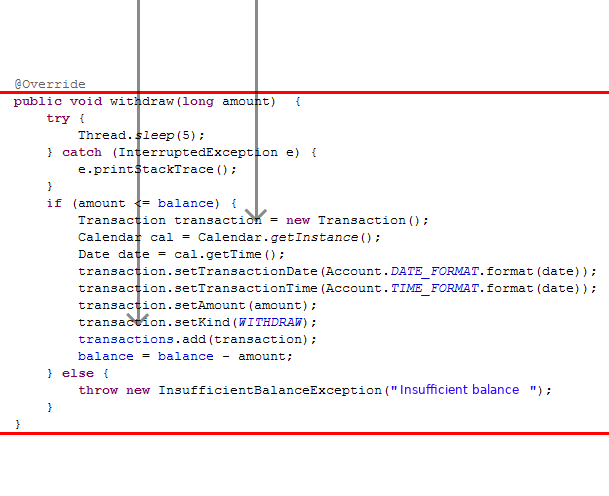Add the synchronized keyword to the withdraw() method to solve the problem. Applying synchronized to a method prevents other threads from entering until the preceding thread leaves the method block.

```@Override
public synchronized void withdraw(double amount)  {
//.. Same as existing code ..
}
```

Test to confirm that there is no longer a negative balance case in the account.
As shown in the figure below, the second thread will not be able to enter the method until the first thread has left the method.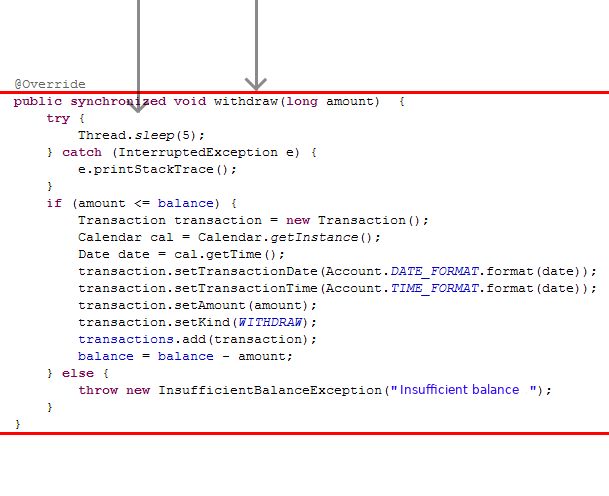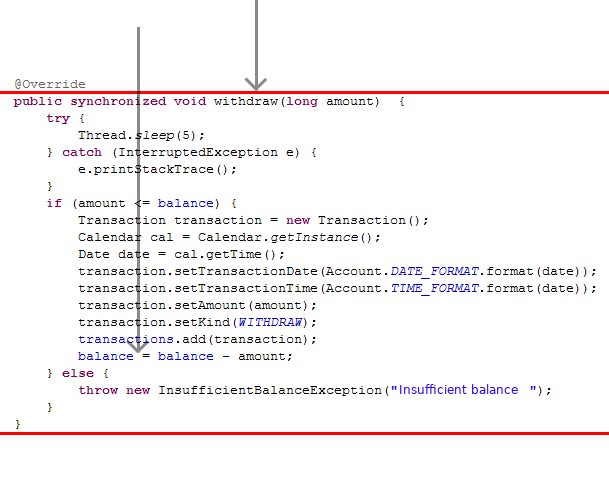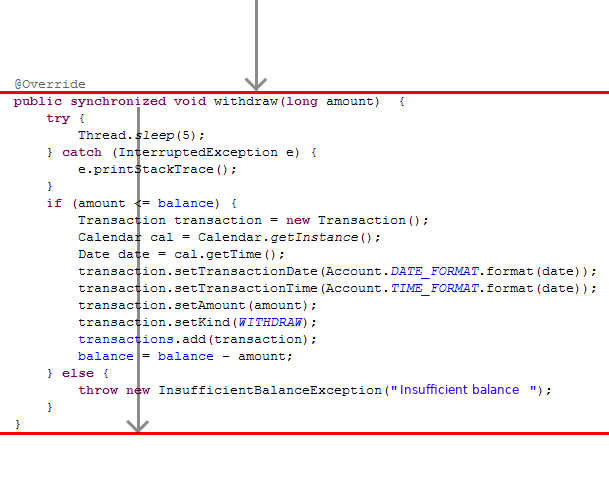When the first thread goes out of the method, the waiting thread enters the method.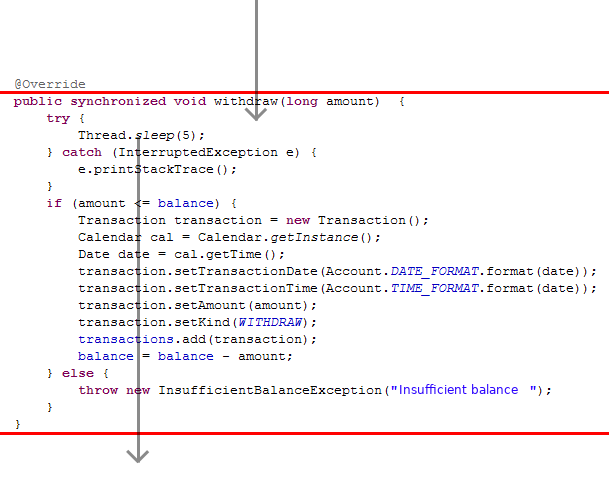Revert the withdraw() method to what it was before modification, as shown below.

```@Override
public synchronized void withdraw(double amount)  {
if (amount > balance) {
throw new InsufficientBalanceException("There is not enough balance.");
}
balance = balance - amount;
Transaction transaction = new Transaction();
Calendar cal = Calendar.getInstance();
Date date = cal.getTime();
transaction.setTransactionDate(Account.DATE_FORMAT.format(date));
transaction.setTransactionTime(Account.TIME_FORMAT.format(date));
transaction.setAmount(amount);
transaction.setKind(Account.WITHDRAW);
transaction.setBalance(this.balance);
```public synchronized void deposit(double amount) {